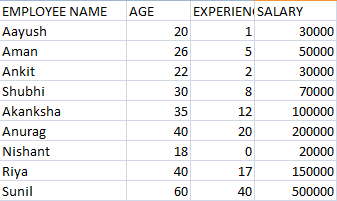# Feature Scaling in Machine Learning using Python

In this tutorial, we will see

• What is Feature scaling in Machine Learning?
• Why is it so important?
• How can we do feature scaling in Python?

In Machine learning, the most important part is data cleaning and pre-processing. Making data ready for the model is the most time taking and important process. After data is ready we just have to choose the right model.

## FEATURE SCALING

Feature Scaling is a pre-processing step. This technique used to normalize the range of independent variables. Variables that are used to determine the target variable are known as features.

#### WHY FEATURE SCALING IS IMPORTANT?

Raw data contains a variety of values. Some values have a small range (age) while some have a very large range (salary). And this wide range can lead to wrong results. Models like KNN and KMeans use Euclidean distance between points for classification and it is very much possible that a feature with large range will influence the results by overpowering other features.

Therefore, we must normalize features before applying certain models. So that the contribution of all features is proportional.

### FEATURE SCALING TECHNIQUES

1. MIN-MAX SCALING
In min-max scaling or min-man normalization, we re-scale the data to a range of [0,1] or [-1,1].2. STANDARDIZATION
In this, we scale the features in such a way that the distribution has mean=0 and variance=1.### PYTHON CODE

DATA SET```import pandas as pd
#importing preprocessing to perform feature scaling
from sklearn import preprocessing
#making data frame
#extracting values which we want to scale
x = data_set.iloc[:, 1:4].values
print ("\n ORIGIONAL VALUES: \n\n", x)
#MIN-MAX SCALER
min_max_scaler = preprocessing.MinMaxScaler(feature_range =(0, 1))
new_x= min_max_scaler.fit_transform(x)
print ("\n VALUES AFTER MIN MAX SCALING: \n\n", new_x)

Standardisation = preprocessing.StandardScaler()
new_x= Standardisation.fit_transform(x)
print ("\n\n VALUES AFTER STANDARDIZATION : \n\n", new_x)
```

OUTPUT

```ORIGIONAL VALUES:

[[    20      1  30000]
[    26      5  50000]
[    22      2  30000]
[    30      8  70000]
[    35     12 100000]
[    40     20 200000]
[    18      0  20000]
[    40     17 150000]
[    60     40 500000]]

VALUES AFTER MIN MAX SCALING:

[[0.04761905 0.025      0.02083333]
[0.19047619 0.125      0.0625    ]
[0.0952381  0.05       0.02083333]
[0.28571429 0.2        0.10416667]
[0.4047619  0.3        0.16666667]
[0.52380952 0.5        0.375     ]
[0.         0.         0.        ]
[0.52380952 0.425      0.27083333]
[1.         1.         1.        ]]

VALUES AFTER STANDARDIZATION :

[[-0.9888666  -0.88683839 -0.68169961]
[-0.50779636 -0.554274   -0.54226105]
[-0.82850985 -0.80369729 -0.68169961]
[-0.18708287 -0.3048507  -0.4028225 ]
[ 0.21380899  0.0277137  -0.19366466]
[ 0.61470086  0.69284249  0.50352812]
[-1.14922334 -0.96997949 -0.75141889]
[ 0.61470086  0.4434192   0.15493173]
[ 2.21826831  2.35566448  2.59510646]]

```

#### WHERE CAN IN WE USE FEATURE SCALING?

1. Linear Regression
In Linear Regression the coefficients are calculated using gradient descent. If we use scaled data, initial random coefficients are closer to the global minima. Therefore, we will find the coefficients in less number of steps.
2. KMeans Clustering AND K Nearest Neighbours
In these methods, Euclidean distance is used, so if one feature has a very large range, it will influence the final results.